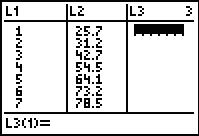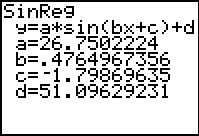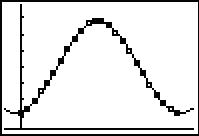Activities

••• Subject Area

• Math: Precalculus: Trigonometry (Triangle and Circular Functions)

• Author9-12

35 Minutes

• Device
• TI-83 Plus Family
• TI-84 Plus
• TI-84 Plus Silver Edition
•TI-84 Plus C Silver Edition
•TI-84 Plus CE
• Software

TI Connect™
TI Connect™ CE

• Report an Issue

Find That SineActivity Overview

Sinusoidal regression is used to determine equations to model various data sets and the equations are used to make inferences.

Key Steps

•Students explore sinusoidal regression by determining and graphing the sine regression equation.

Students will use the program KANSTEMP which will load six lists with data for all three problems.

•They use data for the average monthly temperature for Kansas City to determine the sine equation that models the data.

Students produce a scatter plot with the data given to them in their spreadsheet and find the sine equation that models the data.

•In Problem 2, students will then find the minimum and maximum points on the curve for four dates. These points will represent the summer and winter solstices.